## Склад секції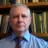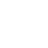Alexander L. Yampolsky Docent (associate professor) of department of fundamental mathematics, z, doctor of sciences in physics and mathematics, docent (associate professor)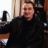Dmytro V. Bolotov Doctor of sciences in physics and mathematics, senior researcher of iltpe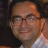Vasyl O. Gorkavyy Doctor of sciences in physics and mathematics, docent (associate professor)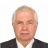. Docent (associate professor) of department of theoretical and applied computer science , phd in mathematics, docent (associate professor)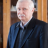P. G. Dolya Docent (associate professor) of department of theoretical and applied computer science , phd for industries

## Schedule for today

 A.L. Yampolsky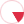Week schedule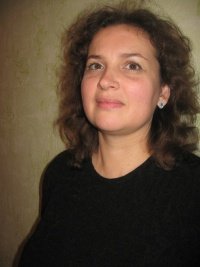# Olena O. Shugailo

Phd in mathematics, senior lecturer

## List of selected publications

Sakharova Ye., Yampolsky A. Powers of Curvature Operator of Space Forms and Geodesics of the Tangent Bundle // Ukr. Math. Journal , 2004. – V. 56, № 9. – p. 1231-1243,

It is well-known that if $\Gamma$ is a geodesic line of the tangent (sphere) bundle with Sasaki metric of a locally symmetric Riemannian manifold then the projected curve $\gamma=\pi\circ\Gamma$ has all its geodesic curvatures constant. In this paper we consider the case of tangent (sphere) bundle over the real, complex and quaternionic space form and give a unified proof of the following property: all geodesic curvatures of projected curve are zero starting from $k_3$, $k_6$ and $k_{10}$ for the real, complex and quaternionic space forms respectively.

Shugailo O. O. On Affine Immersions with Flat Connections // Journal of Mathematical Physics, Analysis, Geometry, 2012. – V.8, № 1. – p. 90-105,

In this paper the multidimensional affine immersions with flat connections of maximal pointwise codimension are studied. The estimates on the dimensions of kernel and the image of the shape (Weingarten) operator and the affine fundamental form are obtained. Some properties of nullity distributions on the immersed submanifold are considered and the examples of affine immersions of high codimension with flat connection are given.

Shugailo O. O. On cylinder representation of affine immersions (russian) // Proc. Intern. Geom. Center, 2012. – 5 (3-4). – p. 68-78,

The multi-dimensional affine immersion with degenerate affine fundamental form is considered. Sufficient conditions under which the nullity is the parallel and affine immersion is a cylinder were found.

Shugailo O. O. Affine Submanifolds of Rank Two // Journal of Mathematical Physics, Analysis, Geometry, 2013. – V.9, № 2. – p. 227-238,

In this paper we study complete connected affine submanifolds $f:M^n \rightarrow \mathbb{R}^{n+m}$ of rank two, i.e. strongly ($n-2)$-parabolic submanifolds by A. Borisenko. We describe the structure of such a submanifold and give an explicit parametrization in two partial cases.

Shugailo O. O. Parallel affine immersion ${M}^n\rightarrow {\mathbb{R}}^{n+2}$ with flat connection (russian) // Ukr. Math. Journal, 2013. – т. 65, № 9. – p. 1283-1300,

We give the description of the parallel affine immersions ${M}^n\rightarrow {\mathbb{R}}^{n+2}$ with flat connection in dependence on the rank of the Weingarten maping.

Shugailo O. O. On affine umbilical immersions of higher codimension (russian) // Proc. Intern. Geom. Center , 2013. – V. 6, № 3. – c. 26-39,

We study the properties of multidimensional affine umbilical immersion with flat connection and locally symmetric connection. We give parametrization of affine umbilical immersion with nilpotent curvature operator.

Shugailo O. O. Affine Curvature of Plane Geodesic Lines on Affine Hypersurfaces (Ukrainian) // Ukr. Math. J., 2017, - V. 69, № 4, - p. 565-574,

We establish a necessary and sufficient condition for a geodesic line on a nondegenerate hypersurface to be a plane curve. We deduce a formula for the affine curvature of a plane geodesic line on the affine hypersurface in terms of the affine fundamental form and the shape operator. We present the definition of transverse curvature and determine some of its elementary properties.